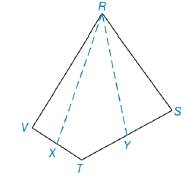Chapter 8.1, Problem 31E### Elementary Geometry for College St...

6th Edition
Daniel C. Alexander + 1 other
ISBN: 9781285195698

#### Solutions

Chapter
Section### Elementary Geometry for College St...

6th Edition
Daniel C. Alexander + 1 other
ISBN: 9781285195698
Textbook Problem
1 views

# For Exercise 31 and 32, X is the midpoint of V T ¯ and Y is the midpoint of T S ¯ . If A R S T V = 48 cm 2 , find A R Y T X .Exercises 31, 32

To determine

To find:

ARYTX If ARSTV=48 cm2

Explanation

“A median of a triangle separated it into two triangles of equal area”.

Calculation:

Given,

Area of RSTV(A)=48 cm2

Draw a diagonal RT¯ in RSTV which separates RSTV into two triangle RVT and RVT

In RVT X is the midpoint of VT¯ from R

(i.e.) RX is the median of RVT since, “A median of a triangle separated it into two triangles of equal area”

(i.e.)RX¯ separates the RVT into RXV and RXT with equal area,

ARXV=ARXT

In RTS,Y is the midpoint of TS¯ from R.

(i.e.) RY¯ is the median of RTS

RY¯ separates the RTS into RYT and RYS with equal area,

ARYT=ARYS

Now, the figure becomes,

### Still sussing out bartleby?

Check out a sample textbook solution.

See a sample solution

#### The Solution to Your Study Problems

Bartleby provides explanations to thousands of textbook problems written by our experts, many with advanced degrees!

Get Started

#### What are the two requirements for a random sample?

Essentials of Statistics for The Behavioral Sciences (MindTap Course List)

#### Factor: 7xy21y

Elementary Technical Mathematics

#### CHECKPOINT 2. If , find the following.

Mathematical Applications for the Management, Life, and Social Sciences

#### True or False: These lines are skew:

Study Guide for Stewart's Multivariable Calculus, 8th

#### True or False: f(t) is used to measure the average rate of change of f with respect to t.

Study Guide for Stewart's Single Variable Calculus: Early Transcendentals, 8th

#### Graph each function. fx=-x2+4

College Algebra (MindTap Course List)